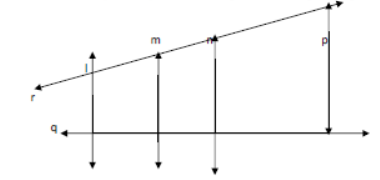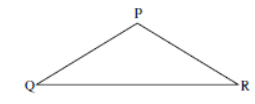# CBSE Class 6 Mathematics Sample Paper Set 7

Read and download PDF of CBSE Class 6 Mathematics Sample Paper Set 7 designed as per the latest curriculum and examination pattern for Class 6 issued by CBSE, NCERT and KVS. The latest Class 6 Mathematics Sample Papers have been provided with solutions so that the students can solve these practice papers and then compare their answers. This will help them to identify mistakes and improvement areas in Mathematics Standard 6 which they need to study more to get better marks in Grade 6 exams. After solving these guess papers also refer to solved Class 6 Mathematics Question Papers available on our website to build strong understanding of the subject

## Sample Paper for Class 6 Mathematics Pdf

Students can refer to the below Class 6 Mathematics Sample Paper designed to help students understand the pattern of questions that will be asked in Grade 6 exams. Please download CBSE Class 6 Mathematics Sample Paper Set 7

### Mathematics Class 6 Sample Paper

CBSE Class 6 Mathematics Sample Paper Set 7.Sample Papers are the very important for every student. The sample papers should be practiced to gain extra marks in examinations. The sample papers have been prepared based on summative assessment1 and summative assessment 2 pattern. The sample papers have been prepared based on pattern of last year examinations and as per latest changes in the syllabus. Students, teachers and parents can download all CBSE educational material and very well prepared worksheets from this website.  All CBSE educational material is developed by our panel of teachers, have also been submitted by CBSE teachers and students.

SECTION – A

Select one correct answer out of the four options given.

1. The smallest odd whole number is

(a) 0 (b) 1 (c) 3 (d) None of these

2. The additive inverse of - 7 is

(a) 0 (b) 1 (c) 7 (d) - 7

3. The complete angle is equal to:

(a) 180o (b) 90o (c) 0o (d) 360o

4. The number of lines passing through two given points is

(a) One and only one (b) two (c) Infinite (d) None of these

5. In Roman Numeral system the symbol M represent:

(a) 100 (b) 500 (c) 1000 (d) None of these

SECTION – B

6. Prove that the number 13856722 is divisible by 11, without actual division.

7. Find the sum of ( - 99 ) + 7 + ( - 101) + 93 using the properties of addition.

8. Write `True or False` for the following

(a) Two lines in the plane always intersect in a point.

(b) Points which lie on the same line are called non-collinear points.

(c) A globe has a curved surface.

9. Draw the following angles

(a) 90o (b) 45o (c) 120o

10. Write any three pairs of parallel line segments in the given figure:SECTION – C

11. Rohan buys 12 computers and 12 printers. If the cost of one computer and one printer is Rs 56233 and Rs 7867 respectively, find the total cost incurred by Rohan( use distributive propert of multiplication)

12. Find the HCF of 204, 144 and 252 by continued division method.

13. Subtract the sum of 38 and – 49 from – 100

14. With the help of a figure, find the maximum and minimum numbers of points of intersection of four lines in a plane.

15. If PQ = 5.4 cm and RS = 2.7 cm, construct a line segment whose length is the difference of the lengths of these line segments.

16. Draw the following using scale and pencil only. Also name them.

(a) One pair of adjacent angles.

(b) One linear pair.

(c) Vertically opposite angles.

(d) One pair of supplementary non-adjacent angles.

SECTION – D

17. Find the least number that should be added to 2000 so that 45 divides the sum exactly.

18. Find the least number which when divided by 40,50, and 60 leaves remainder 5 in each case.

19. Sum of two integers is 48. If one of them is – 25, find the other.

20. Construct a line segment whose length is equal to the sum total of all the three sides of this triangle. Also write the length of all the sides.21. Write the kinds of angle formed between the following directions:

(a) North and South.

(b) North and North west.

(c) South and East.

(d) East and west.

(e) East and East.

Please click the below link to access CBSE Class 6 Mathematics Sample Paper Set 7.